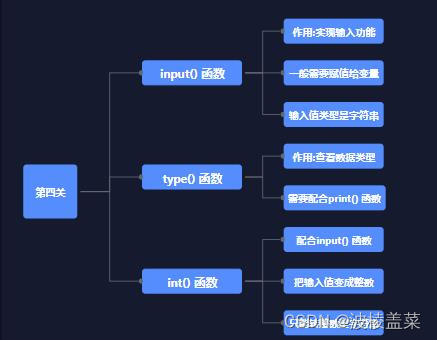### 一、input（）函数

``name=input('请从 ABCD 中选择你要娶的姑娘：') print(name) #D``

### 二、input（）赋值

``answer = input('请从 ABCD 中选择你要娶的姑娘：')``

``answer = input('请从 ABCD 中选择你要娶的姑娘：')print(answer)``

### 三、input（）类型

``answer = input('秋香的生日是8月几号？') if answer == 15:   print('答对了，我同意这门亲事') else:   print('生日都说错了？秋香不能嫁给你')``

`if` 语句中的判定条件是 `answer == 15`，注意，右端的 `15` 是一个整数，所以，进入 `if` 分支的条件就是：变量 `answer` 中储存的数据等于 整数 `15`

``price =int(input('请输入冰糖葫芦的价格：')) if price < 15:   print('买一根吧') else:   print('不买了') #输入15 #输出15``